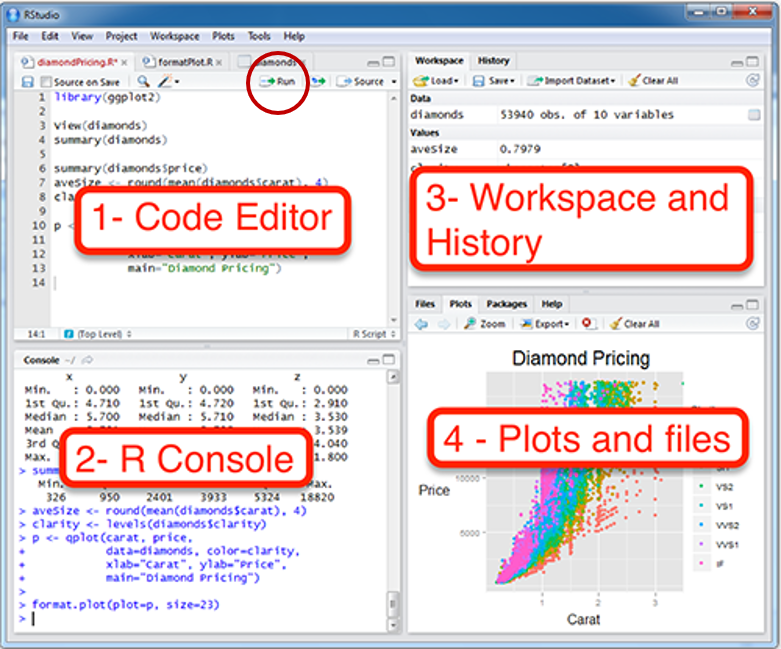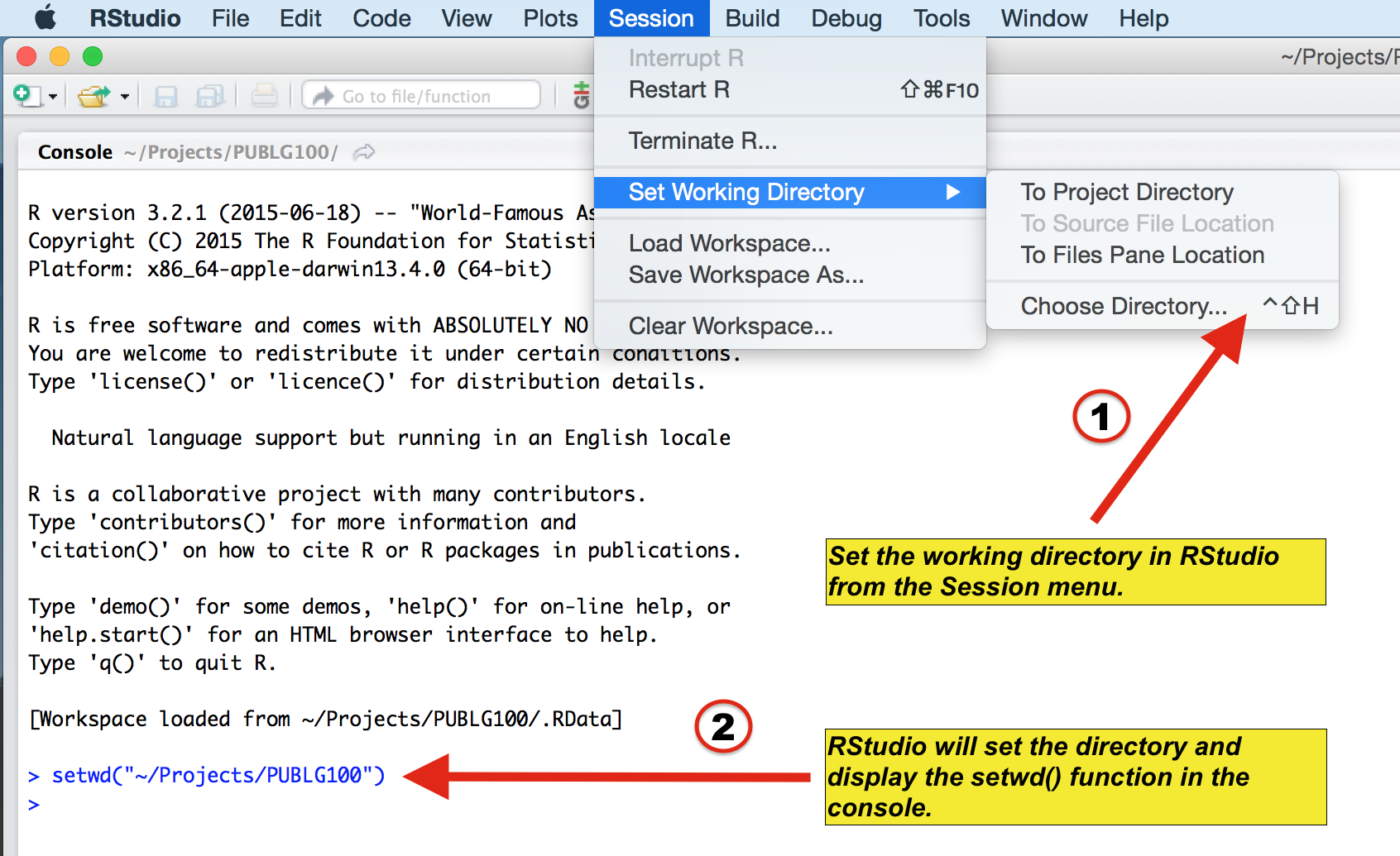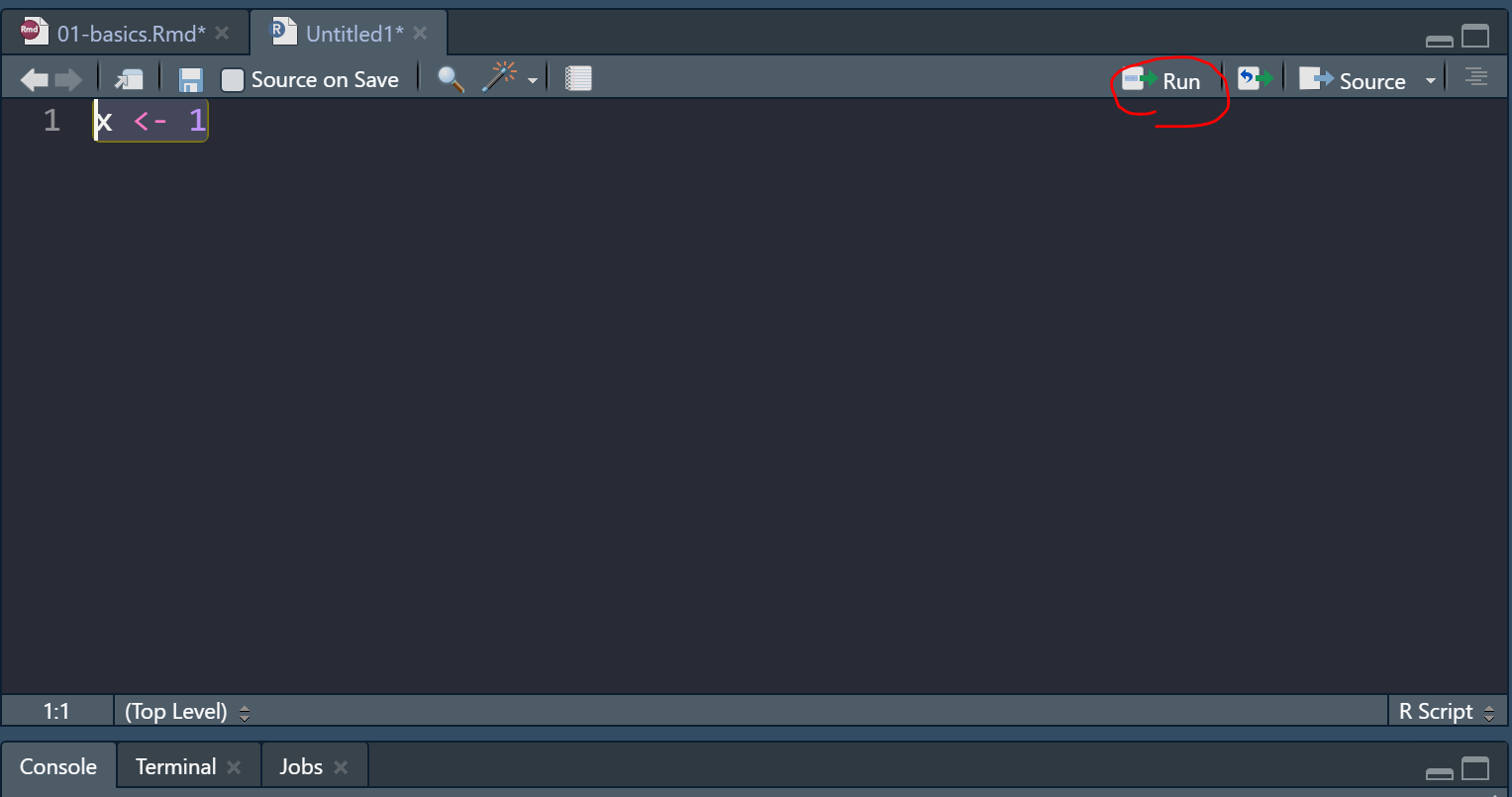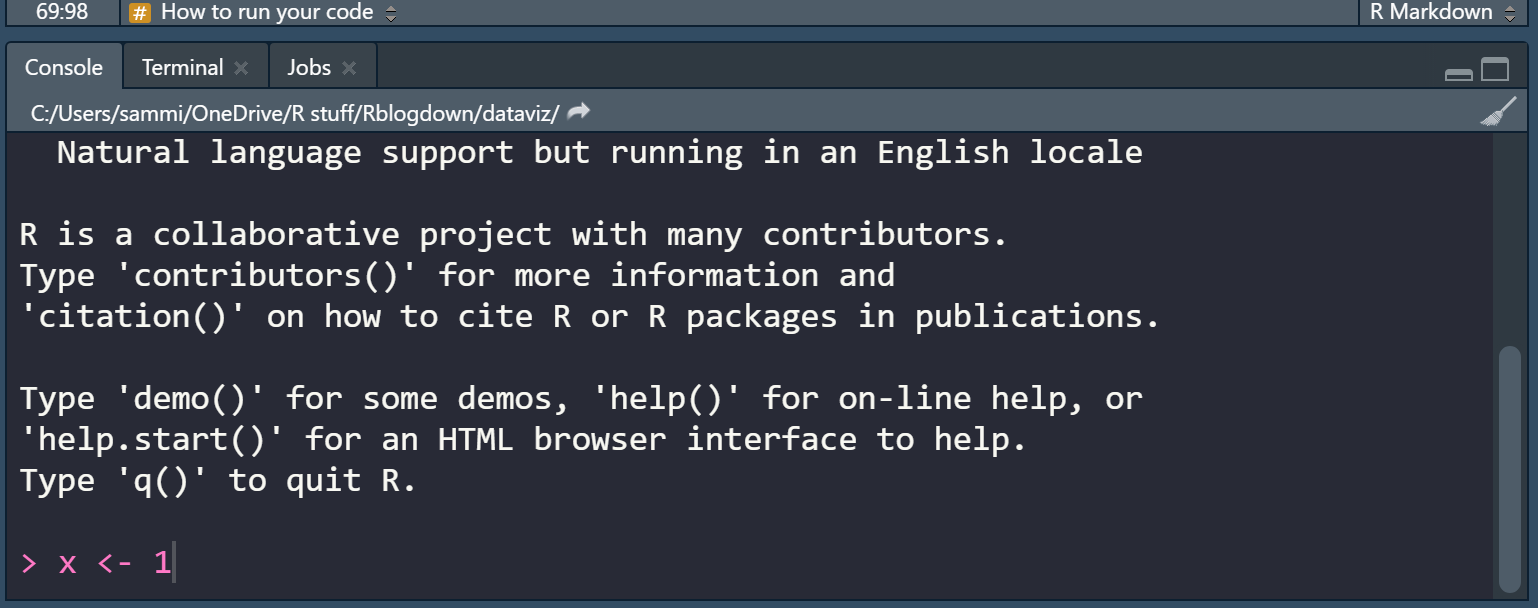## 1.1 Setting up RStudio

### Why use R for data visualization?

• R is free.
• Often, less codes are needed in R to plot an elegant graph.
• for/while loops (basic concepts in programming) are not necessary in R to make a production-quality graph.
• R is the best software for statistical analysis.

### Open RStudioFigure 1.1: Rstudio layout, image from http://www.sthda.com/english/wiki/running-rstudio-and-setting-up-your-working-directory-easy-r-programming

• Rstudio is where people do R programming.
• You can type codes (commands) into the console (bottom-left panel).
• > means that the console is ready to receive more code.
• + means your code is not complete.
• You can also write (longer) codes in the script within the code editor (top-left panel).
• The code editor will run the script into the console.
• A new script can be opened by clicking: File -> New -> R Script.
• You can run a script by clicking ’Run” with the green arrow or by typing ctrl + enter. It is labeled with the red circle.
• Or you can just type your codes directly into the console.

### Let’s make a folder and set it as working directoryFigure 1.2: Setting your working directory, image from https://www.ucl.ac.uk/~uctqiax/PUBLG100/2015/faq/setwd.html

### 1.1.1 How to run your code

• You can run code in 2 ways.

• First, you can type it in the script and run it after highlighting the codes you would like to run.Figure 1.3: How to run your code - script

• Second, you can type your code directly in the console.Figure 1.4: How to run your code - console

## 1.2 Basics of R

### Let’s type some codes

• Capitalization, punctuations and brackets are all important.
• ' ' and " " mean the same.
• However, ' " are not paired. So they will not work.
• = and <- mean equivalent.
• I often use <-.
• Type ? when you are not sure about the code (ex. ?t.test)
• A code becomes comment when it is preceded by #.
• Try typing # g = 3 and see if the number gets stored in g by typing g in the console.
x = 3 # x equals to 3
a <- 4 # a equals to 4
d <- 'Group' # gr is equal to a character 'Group', which is not a number.
e <- "Group"
d == e # 'Group' and "Group" are equal
##  TRUE
# g = 3 # its a comment
• Notice that I used == to test if d and e are equal. Therefore, == and = mean different. == tests if two things are equal. = sets two things to be equal.
• d == e returns TRUE because they are both 'Group'. TRUE is equivalent to 1 numerically.
• Now let’s check if x and a are equal.
x == a
##  FALSE
• It returns FALSE because x and a are not equal. This is correct because 3 and 4 are not equal. FALSE equals to 0 numerically.

• Below are more examples showing that TRUE = 1 and FALSE = 0.

TRUE + FALSE # 1 + 0
##  1
TRUE + TRUE # 1 + 1
##  2
FALSE + FALSE # 0 + 0
##  0
TRUE*2 # 1 * 2
##  2
TRUE*FALSE # 1 * 0
##  0

### How can I learn most effectively with the notes?

• Don’t just copy and paste the codes and run them in RStudio (ctrl + c & ctrl + p). Make sure you type each code.
• You can also change your code and see if it still works.
• If you are not sure of your code, you can type ? before the function.
• If you are still not sure after reading the notes, check out Chapter 3 of R for Data Science: https://r4ds.had.co.nz/

### More installation

• R is an old programming language.
• So, people, such as statisticians and programmers, have created more functions in R in the form of the package to update the language. They are free but they have to be downloaded separately.
• A package can contain several functions.
• In this tutorial, you will mainly be using ggplot2 package, which is elegant and flexible for visualizing data.
• Also, you will be using smplot package. It improves ggplot2 graphs visually.
• So, you will need to install some packages, such as ggplot2 and smplot. Please type the codes below.
install.packages('devtools')
devtools::install_github('smin95/smplot', force = TRUE) # requires VPN if you are in China
• You only need to install them once, but you will need to reload them whenever you start a new session in RStudio using the function library().
install.packages('tidyverse') # only need to install once
install.packages('cowplot')
# packages must be loaded every time by using library() when you run your script

library(tidyverse) # it has ggplot2 package
library(cowplot) # it allows you to save figures in .png file
library(smplot)
• Now let’s make some graphs in the subsequent chapters.